# Cost Function in MATLAB

December 24, 2021

By  Sneha

lang: en_US

Are you working on supervised learning and searching for a method to reduce the error? And do you want to find better values for parameters? Then this blog is for you.

You can reduce errors and can give better values for parameters using cost function and gradient descent. So, without wasting precious time, Let’s discuss these concepts and solutions for these problems.

### Introduction:

Before discussing the actual topic, Let’s understand “Hypothesis(h)”. Hypothesis is a function which predict values(h) with given input values(x). For example, An ML (Machine learning) algorithm has to be built to predict the cost price of selling a house, given the size of the site and training data. (This example is considered throughout this blog to understand the concept).Training data with size and price of the rent house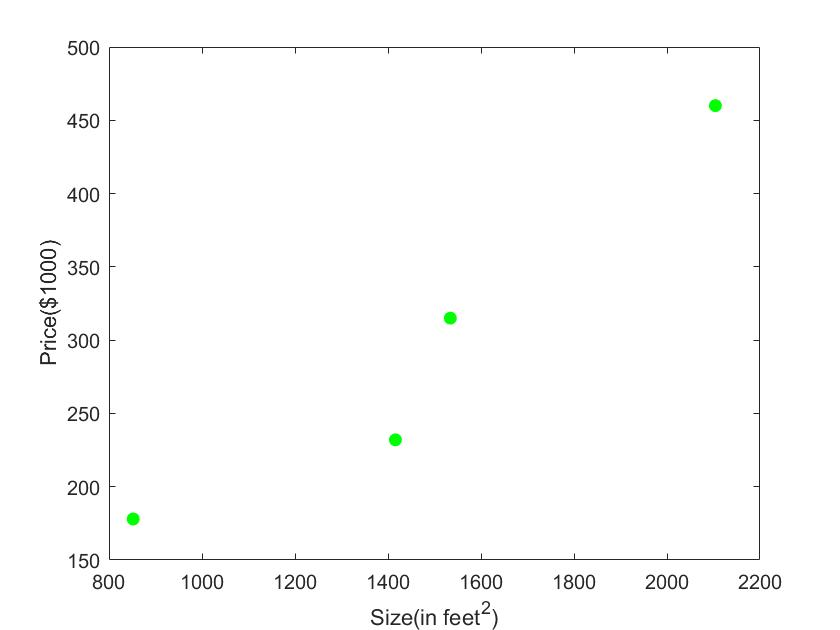Plot between size and price of the house.

Now we need to generate a hypothesis function which is denoted by ‘h(x)’. It is a polynomial function that takes one or more parameters. For example: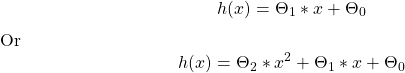It applies to all polynomial functions. For every input value (x), it predicts output using hypothesis function (h). The general form of hypothesis function is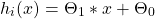where i=0,1,…..,N

Therefore ‘h’ is a vector of predicted values for an algorithm.

Our objective is to get the best possible line. The best possible line will be such that the average squared vertical distances of the scattered points from the line will be the least. Ideally, the line should pass through all the points of our training data set. In such a case, the value of cost function (J) will be zero.  For the example mentioned above, Let’s consider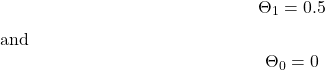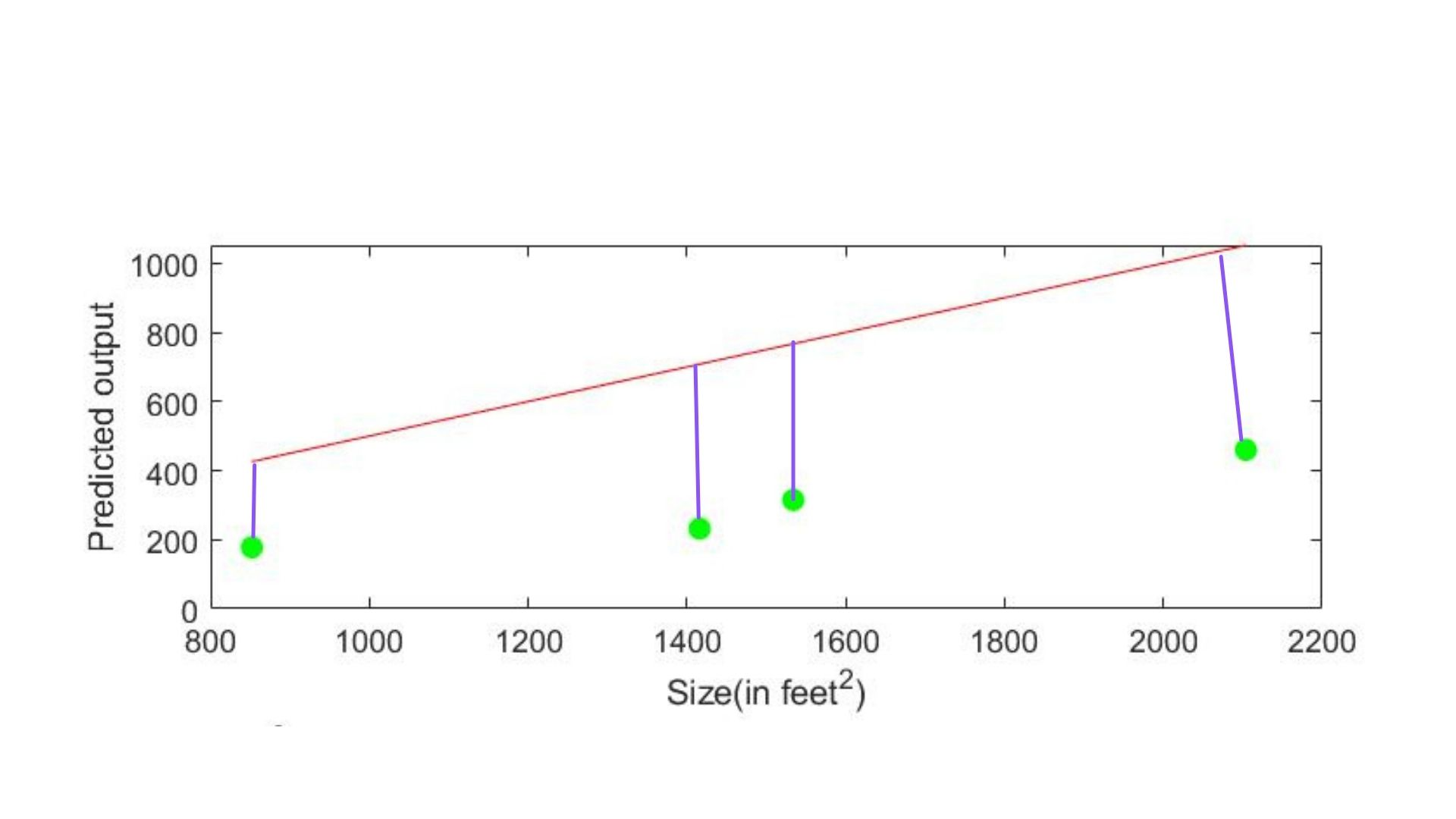The plot of comparison between predicted output and actual output. The Red line shows the hypothesis function, and green circles are the actual output.

In the above figure, You can observe that the actual values (y, output of training data) and predicted values (h) are different and have some differences. So, To make the algorithm optimum, we need to reduce this difference during training. And this difference itself is called “Cost function”. The method used to reduce cost function is called “Gradient descent”.

## Cost Function:

It is the average difference of all the results of the hypothesis (h) and the actual output (y). The cost function is denoted by “J”. This is also called as “Squared Error function” or “Mean square Error”. The mathematical equation is given by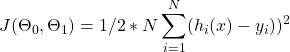Where  N-->Number of training data.

h-->Predicted output

y-->Actual output

The mean is halved as a convenience for computation of the gradient descent as the derivative term of the square function will cancel out the halved term.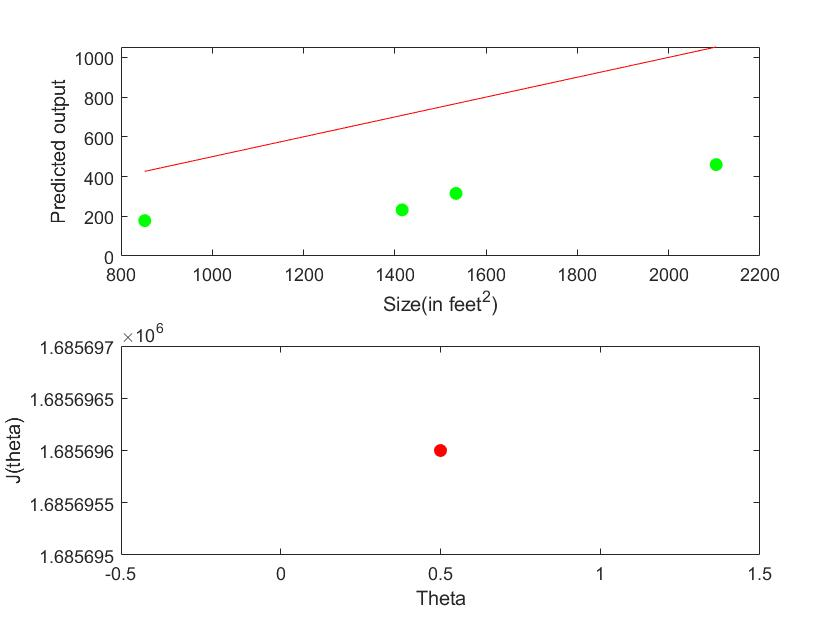Cost function graph with theta=0.5.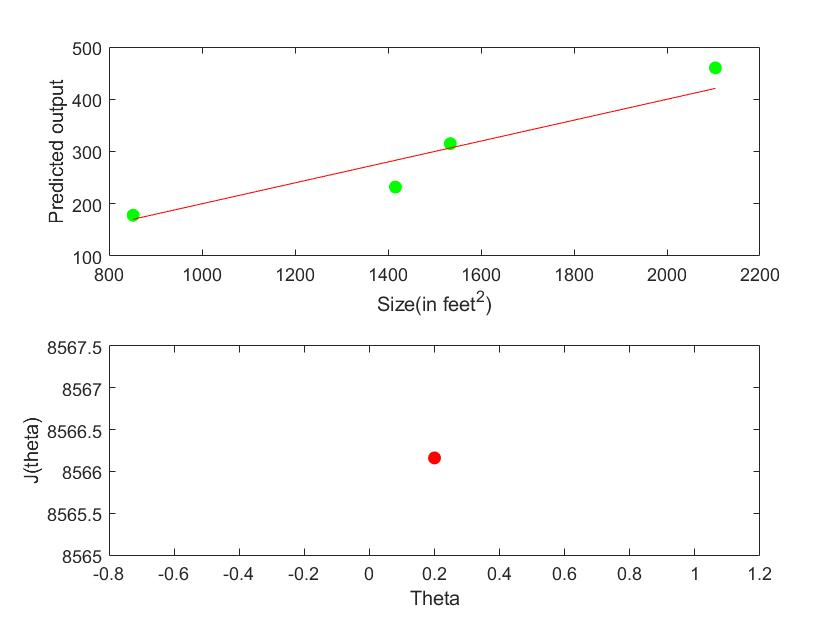Cost function with theta=0.2

So from the above example, you understood that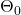and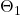are values that play an important role in prediction. Thus we have to improve thisandvalues, such that the cost function reaches its optimal minimum. Therefore, the Gradient Descent algorithm is best to attain this feature.

It is an optimization approach for determining the values of a function’s parameters that minimizes a cost function. It is a derivative (the tangential line to the function) of the cost function. The slope of the tangent is the derivative at that point and it will give us a direction to move towards. We make steps down the cost function in the direction with the steepest descent. The size of each step is determined by the parameter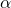, which is called the “learning rate”.

For example, the distance between each “star” in the graph above represents a step determined by our parameter. A smallerwould result in a smaller step, and a largerresults in a larger step. The direction in which the step is taken is determined by the partial derivative of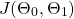.

The gradient descent algorithm is given by:

Repeat until convergence: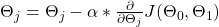But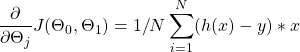Therefore, Repeat until convergence:Where j= 0,1 represents the feature index number

At each iteration j, one should simultaneously update the parameters. Updating a specific parameter before calculating another one on the jth iteration would yield a wrong implementation.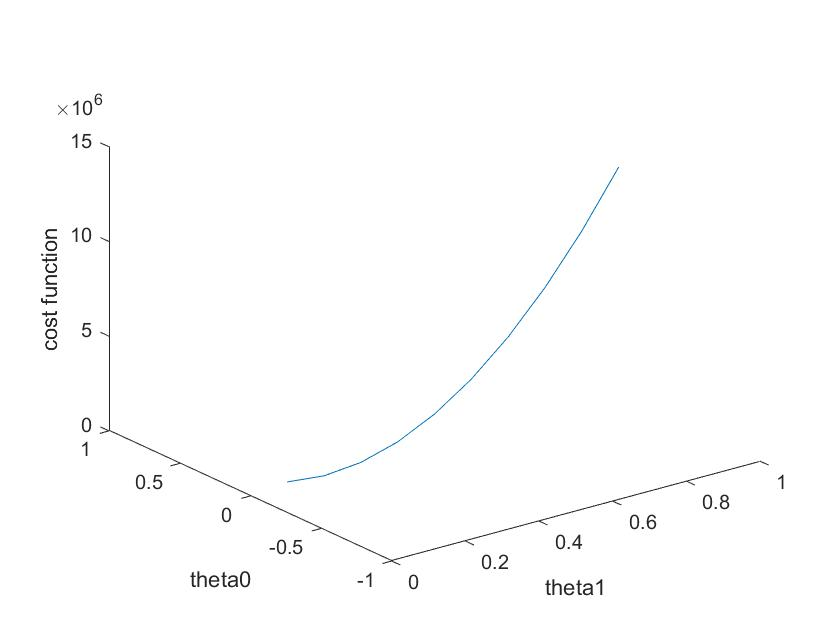3D plot of Cost function to determine the optimal minimum (at 0.2,0) with respect to the cost function.

Here our objective is to converge the cost function to its minimum value. This convergence depends on the parameter “”.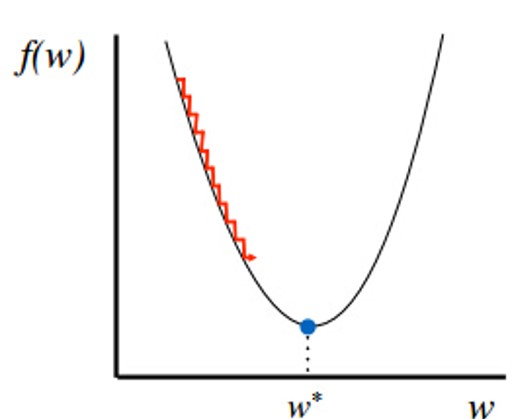Plot of optimization of theta value when alpha is too small.

If “” is very small, gradient descent can be slow (It may take a long time to reach its minimum).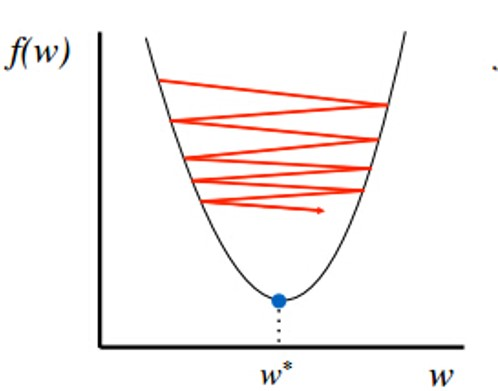Plot of optimization of theta value when alpha is very large.

If “” is too large, gradient descent Can overshoot the minimum. It may fail to converge or even diverge.

### MATLAB CODE:

%%%%%%%%%%%%%%%%%%%%%%%%%%%%%%%%%%%%%
% Author:Sneha G.K
% Topic :Cost Function in MATLAB
% Company: MATLAB Helper
% Website: https://MATLABHelper.com

%%%%%%%%%%%%%%%%%%%%%%%%%%%%%%%%%%%%%
% MATLAB code to find and improve parameter(theta value).
clc;
clear;
close all;
% Training data size of house(x) and price of house(y).
x=[2104 1416 1534 852];
y=[460 232 315 178];
%Theta value to be inserted in hypothesis function.
%Theta value can be 1x1 scalar or nx1 vector.
theta=input("Enter the theta1 value:");
N=length(y);
h=theta*x;%hypothesis fuction.
% Formula to find cost function.
Error=(h-y).^2;
J=(1/(2*N))*sum(Error);
disp(J);
% alpha to find gradient descent.
alpha=input("Enter alpha value:");
% Numbaer of times the parameters to be updated.
num_iter=input("Enter the number of times the parameter need to be updated:");
% The logic behind the gradient descent.
% If parameters are nx1 vector then another for loop has to inserted to
% updated every theta value.
for i=1:num_iter
Error=h-y;
delta=x'*Error;
theta=theta-(alpha/N)*sum(sum(delta));
h=theta*x;endError=(h-y).^2;
J=(1/(2*N))*sum(Error);
disp(J);
% display theta value
subplot(2,1,1);
plot(x,y,"ro","MarkerFacecolor","r");
hold on;
plot(x,h,"g-");
xlabel("Size(in feet^2)");
ylabel("Predicted value");
hold off;
subplot(2,1,2);
plot(theta,J,"bo","MarkerFacecolor","b");

### Conclusion:

• The cost function is used to determine the quantity of error in prediction, which uses training data.
• Cost function plays an essential role in training the ML (Machine Learning) algorithm.
• A gradient descent algorithm is used to reduce the cost function for regression.

Ready to take your MATLAB skills to the next level? Look no further! At MATLAB Helper, we've got you covered. From free community support to expert help and training, we've got all the resources you need to become a pro in no time. If you have any questions or queries, don't hesitate to reach out to us. Simply post a comment below or send us an email at [email protected].

And don't forget to connect with us on LinkedIn, Facebook, and Subscribe to our YouTube Channel! We're always sharing helpful tips and updates, so you can stay up-to-date on everything related to MATLAB. Plus, if you spot any bugs or errors on our website, just let us know and we'll make sure to fix it ASAP.

Ready to get started? Book your expert help with Research Assistance plan today and get personalized assistance tailored to your needs. Or, if you're looking for more comprehensive training, join one of our training modules and get hands-on experience with the latest techniques and technologies. The choice is yours – start learning and growing with MATLAB Helper today!

Education is our future. MATLAB is our feature. Happy MATLABing!

#### Sneha

I am Sneha G.K . I am an Electronic and Communication Engineering Student. I am a passionate Programmer and a researcher too. I enjoy exploring new things everyday and experimenting it.

•David Barnwell says:

How do you determine the number of iterations? Is it the same as the size of the data set? I’m assuming theta is the straight line gradient? Is alpha the intercept value of the regression that’s been previously calculated?

•Sneha G.K says:

1.It is a great question but unfortunately number of iteration value is like trial and error, you can try for some set of values till you reach your destination.This is the main reason why we opted neural network.
2.No, it depends on the theta value you give at initial stage.
3. Yes alpha is intercept for previously calculated regression.

•David Barnwell says:

Very nice blog..but this is my question. I’m unclear about the source of numiter, theta and alpha. I;m thinking that you could use least squares algorithm to generate a model. Then, you could use the gradient of the model as an estimate for theta. The size of the data set could be used an estimate for numiter and finally, the intercept of the model could be used as an estimate for alpha in descent gradient. Am I right?

•Sneha G.K says:

You can do that. But numiter doesn’t depend on dataset.It depends on the theta value you provide at initial code. Intercept can be used as alpha for Gradient descent.

#### MATLAB Helper ®

Use Website Chat or WhatsApp at +91-8104622179